Science, Maths & Technology

### Become an OU student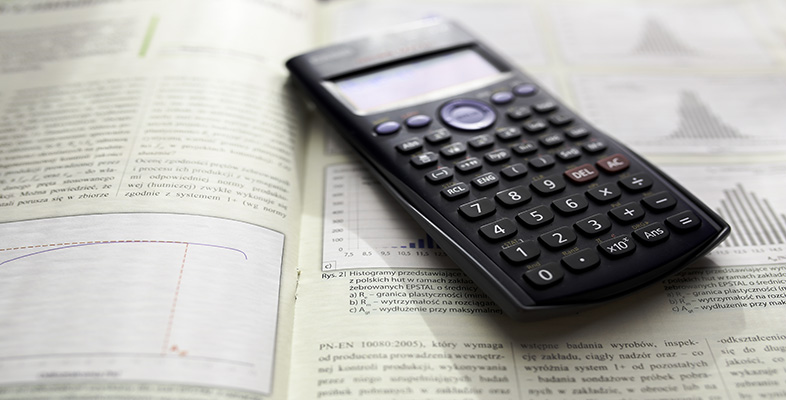Using a scientific calculator

Start this free course now. Just create an account and sign in. Enrol and complete the course for a free statement of participation or digital badge if available.

# 1.1 Basic calculations

Basic calculations are entered into the calculator in exactly the same order as they are written on paper, as demonstrated in the following activity. The calculator displays the calculation that you enter. When you press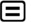, the answer is displayed at the bottom right of the screen.

## Activity 2 Sums, differences, products and quotients

Use your calculator to work out the answers to the following calculations.

1.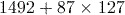2.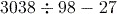3.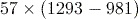4.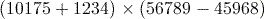1.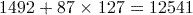Note that the calculator uses the BIDMAS rules. These say that any expression within Brackets should be calculated first, then any Indices (often called powers), followed by Divisions and Multiplications and finally Additions and Subtractions.

2.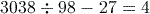3.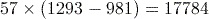To calculate this correctly you need to remember to insert the brackets into the calculation using the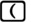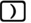keys.

4.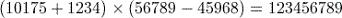You may have noticed that in part (4) of the above activity, the calculation was too long to fit on the calculator display. In such circumstances, the symbols ‘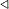’ or ‘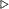’ appear at the left or right of the display to indicate that there is more information in that direction. This information can be seen by scrolling left or right using the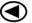and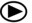keys, which are found at the left and right sides of the large cursor control button (labelled with the word ‘REPLAY’) located under the calculator screen.

If you type a very long calculation into your calculator,  then you may see the cursor  (which is usually shown as ‘’) change to ‘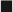’. This means that you are allowed to type only at most 10 more characters. If you encounter this, you should break your calculation into smaller parts.

The cursor is a flashing symbol indicating where the next item entered into the calculator will appear.